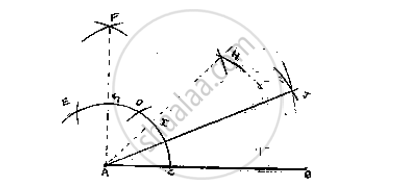# Construct the Angle of the Measurement: 1. 22 (1°)/2 - Mathematics

Construct the angle of the measurement:

1. 22 (1°)/2

#### SolutionSteps of construction:
Step 1: Draw a line segment AB
Step 2: with center A and any radius, draw an arc which intersects AB at C
Step 3: with center C and same radius, draw an arc which intersect previous arc at D
Step 4: with center D and same radius, draw an arc which intersects arc in (2) at E.

Step 5: with center E and D and radius more than half of ED, draw arcs intersecting each other at F.
Step 6: Join FA which intersects arc in (2) at G
Step 7: with center G and C and radius more than half of GC, draw arcs intersecting each other at H
Step 8: Join HA which intersects arc in (2) at 1.
Step 9: with centers I and C and radius more than half of IC, draw arcs intersecting each other
Step 10: Join JA
∴ ∠JAB = 22 (I°)/2

Concept: Basic Constructions
Is there an error in this question or solution?

#### APPEARS IN

RD Sharma Mathematics for Class 9
Chapter 16 Constructions
Exercise 16.2 | Q 11.6 | Page 9

Share